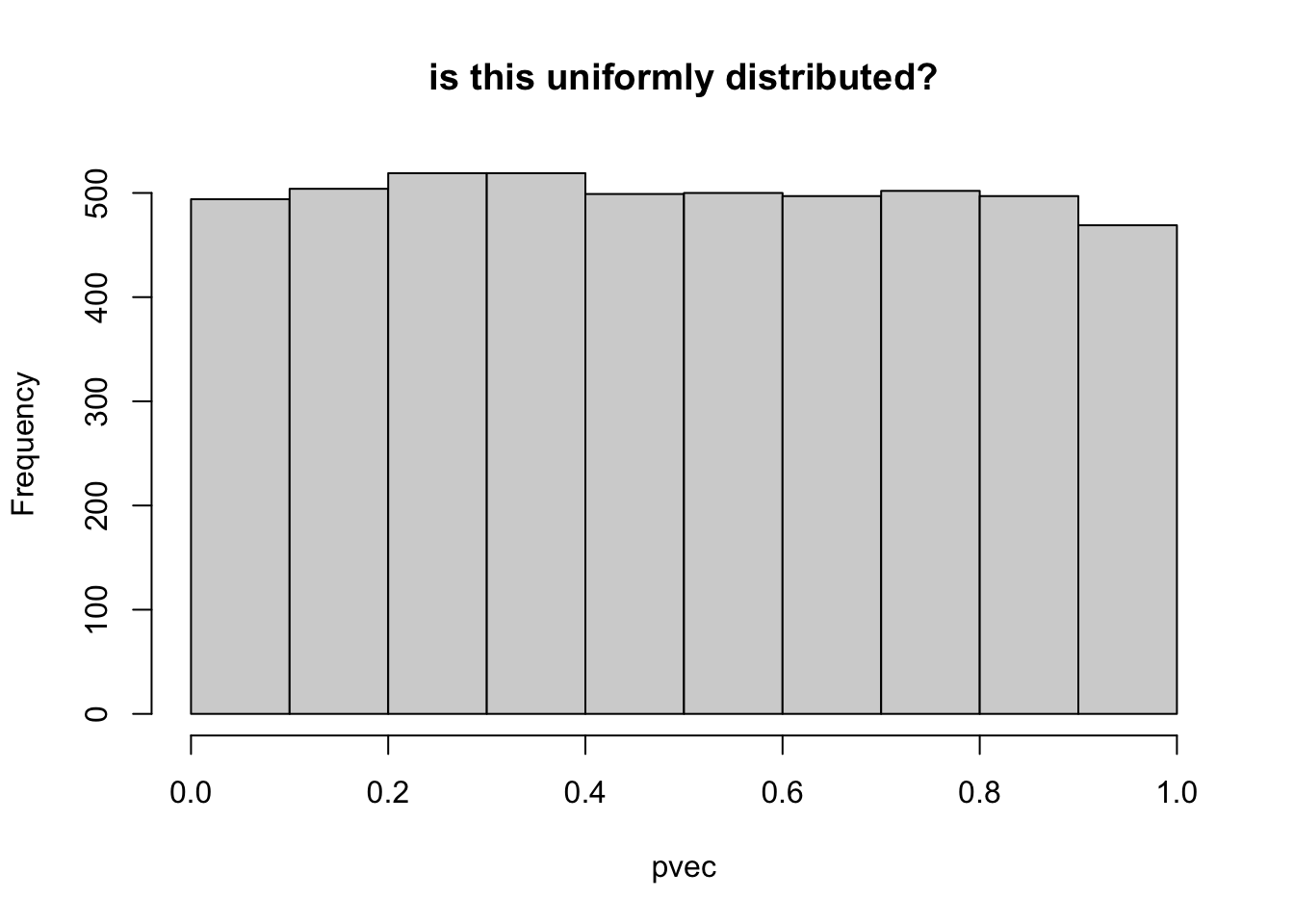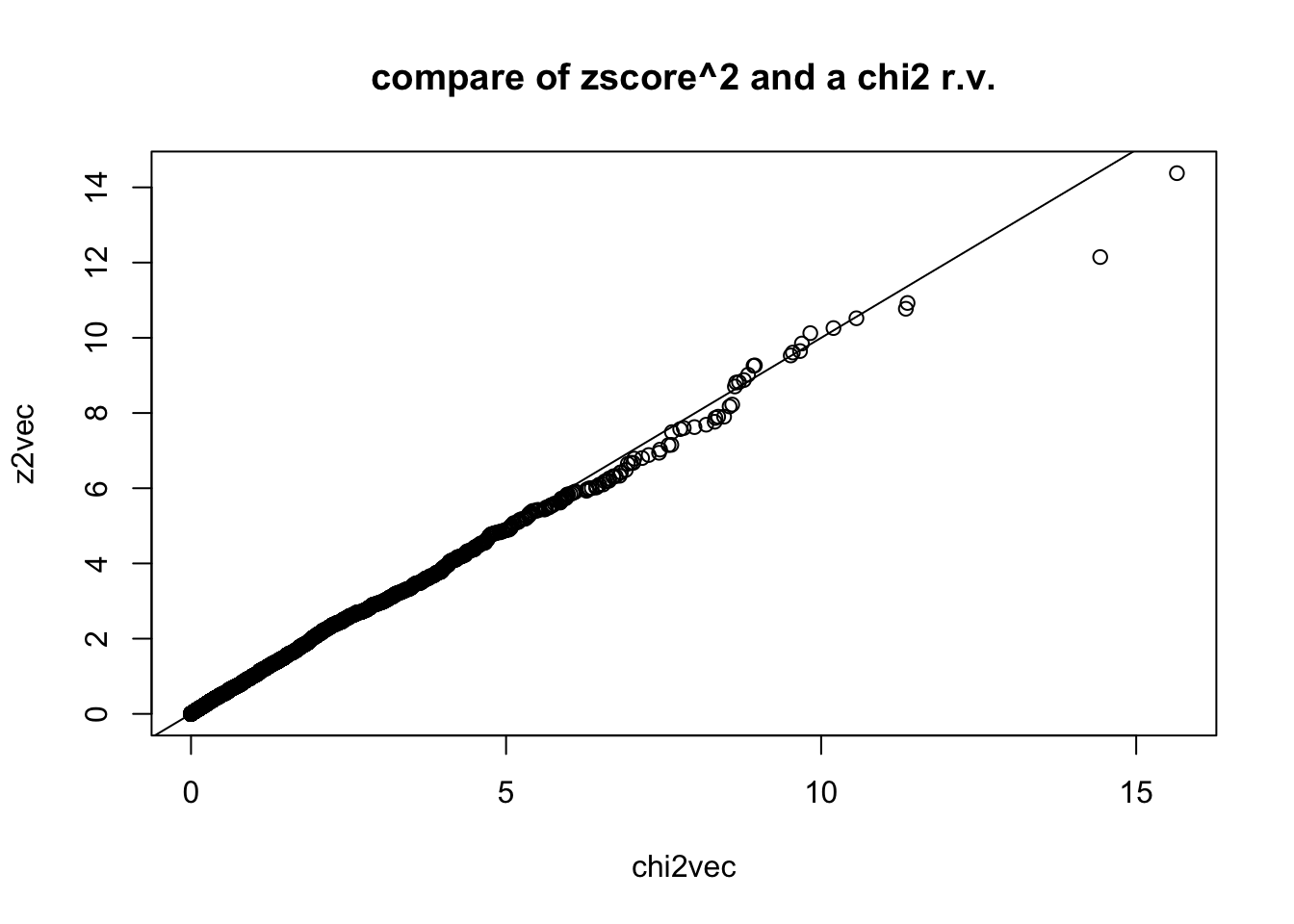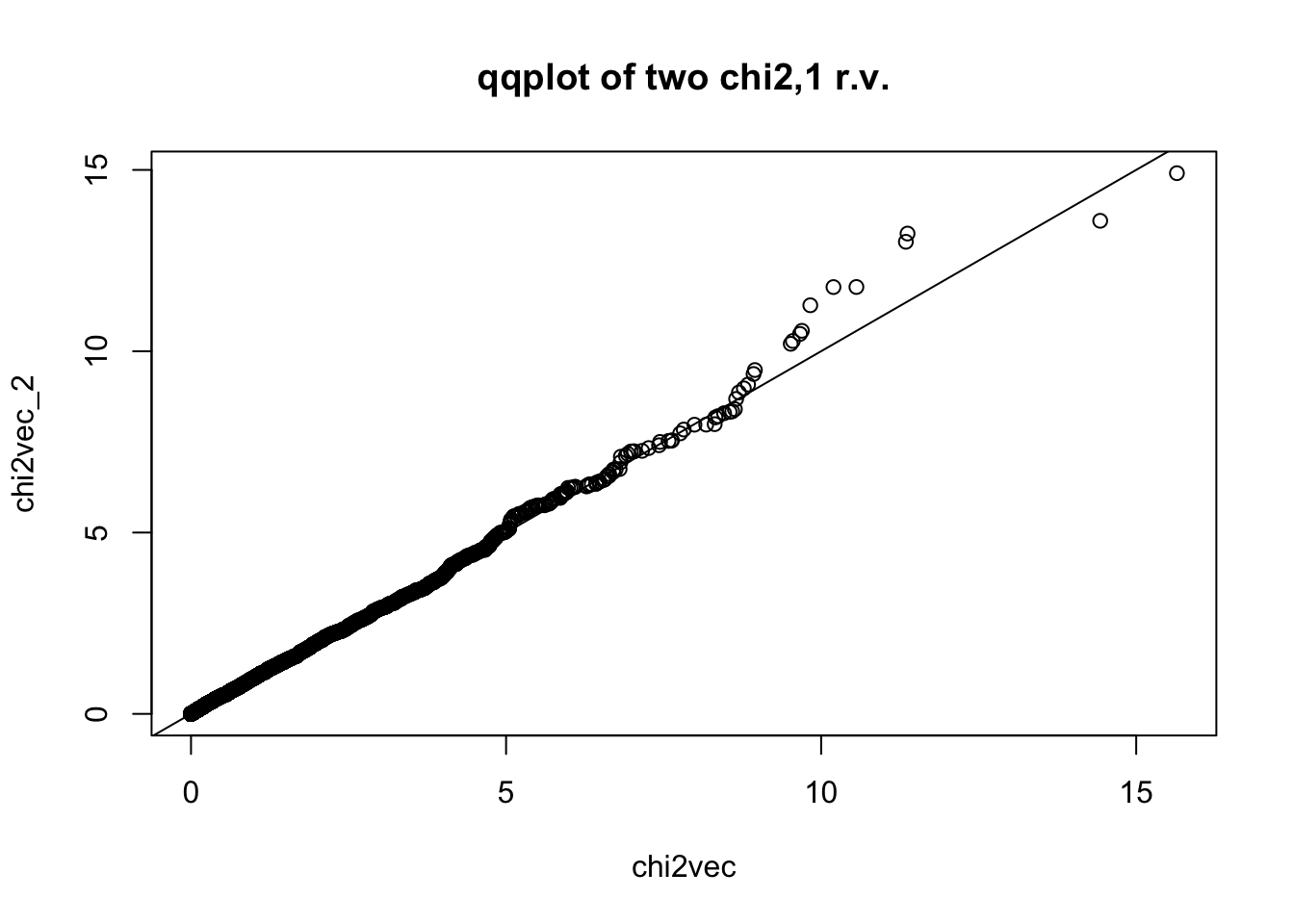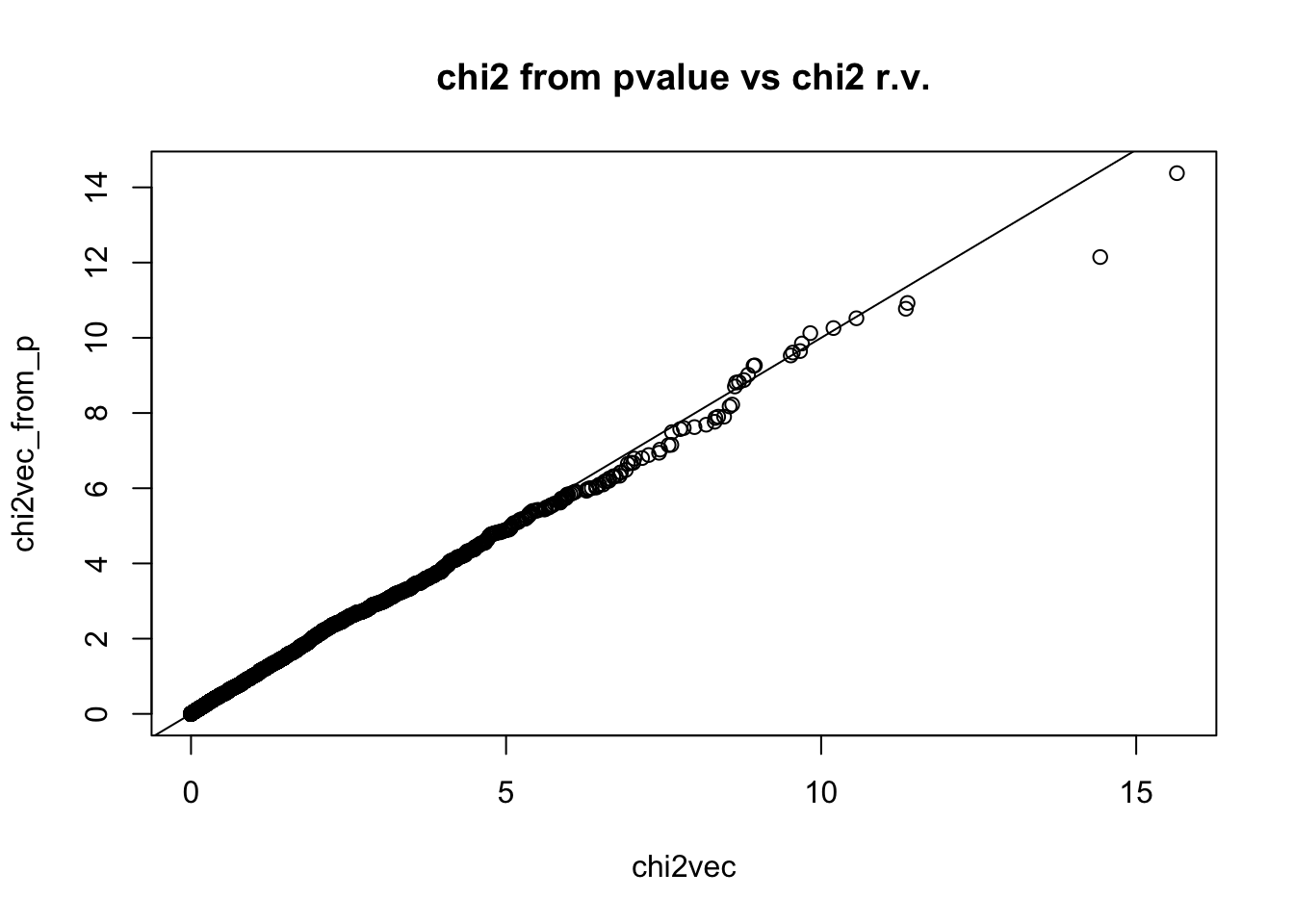# How to calculate Z-score, P-value, Chi2 stat from GWAS

Author

Haky Im

Published

April 13, 2022

Summary

Given one of the statistics in a GWAS (Z-score, P-value, or chi2), calculate the others.

## Calculate Zscore, p-value, Chi2 statistics using GWAS summary statistics

$Y = \beta \cdot X + \epsilon$ GWAS summary statistics will contain an estimate of the regression coefficient $$\hat\beta$$ and its standard error $$\text{se}(\hat\beta)$$ for each SNP in the GWAS.

We distinguish the true $$\beta$$ from the estimated value $$\hat\beta$$ using a hat.

## Z-score

$Z = \frac{\hat\beta}{\text{se}(\hat\beta)}$ $Z \approx N(0,1) ~~~~~~ \text{as } n \rightarrow \infty$

## Z to Chi2 statistic

$Z^2 \sim \chi^2_\text{df=1}$

Under the null, the squared of the Z-score follows a $$\chi^2$$ distribution with 1 degree of freedom.

## Z to P-value

$P = \text{pnorm}(-|Z|) * 2$

## P to Z

From the p-value, we can calculate the magnitude of the Z-score but the sign is lost. So the Z-score has more information than the p-value.

$|Z| = |\text{qnorm}(P/2)|$

## P to Chi2

$\chi^2 = \text{qnorm}(P/2)^2$

## Simulations

Let’s simulate zscore vector under the null hypothesis.

Show the code
nsim = 5000
set.seed(2021021001)
zvec = rnorm(5000, mean=0, sd=1)

Calculate the p-value (probability that a normal r.v. will be as large or larger in magnitude than the |zscore|

Show the code
pvec = pnorm(-abs(zvec)) * 2 ## two-tailed
## check pvec is uniformly distributed
hist(pvec,main="is this uniformly distributed?")Show the code
## remember that if square the a normal r.v. you get chi2 r.v. with one degree of freedom
z2vec = zvec^2
## compare with chi2 rv. with 1 degree of freedom by simulating chi2,1 and qqplot
chi2vec = rchisq(nsim,df=1)
qqplot(chi2vec,z2vec,main="compare of zscore^2 and a chi2 r.v."); abline(0,1)Show the code
## test whether the distributions of z2vec and chi2vec are different using the Kologorov Smirnov test
ks.test(z2vec,chi2vec)

Asymptotic two-sample Kolmogorov-Smirnov test

data:  z2vec and chi2vec
D = 0.019, p-value = 0.3275
alternative hypothesis: two-sided
Show the code
## for reference, let's compare two chi2,1 r.v.'s qqplot
chi2vec_2 = rchisq(nsim,df=1)
qqplot(chi2vec,chi2vec_2,main="qqplot of two chi2,1 r.v."); abline(0,1)Show the code
ks.test(chi2vec,chi2vec_2)

Asymptotic two-sample Kolmogorov-Smirnov test

data:  chi2vec and chi2vec_2
D = 0.0158, p-value = 0.5605
alternative hypothesis: two-sided

Sometimes you get the p-value instead of the zscore, you can generate chi2 by inverting the relationship.

Show the code
chi2vec_from_p = qnorm(pvec / 2)^2
qqplot(chi2vec,chi2vec_from_p,main="chi2 from pvalue vs chi2 r.v."); abline(0,1)